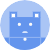search
Search
Publish
Guest 0reps
Thanks for the thanks!
close
account_circle
Profile
exit_to_app
Sign out
search
keyboard_voice
close
Searching Tips
Search for a recipe: "Creating a table in MySQL"
Search for an API documentation: "@append"
Search for code: "!dataframe"
Apply a tag filter: "#python"
Useful Shortcuts
/ to open search panel
Esc to close search panel
to navigate between search results
d to clear all current filters
Enter to expand content previewDoc SearchCode Search BetaSORRY NOTHING FOUND!
mic
Start speaking...Voice search is only supported in Safari and Chrome.
Shrink
Navigate to
A
A
share
thumb_up_alt
bookmark
arrow_backShareTwitterFacebook

# NumPy | mean method

Programming
chevron_right
Python
chevron_right
NumPy
chevron_right
Documentation
schedule Mar 9, 2022
Last updated
local_offer PythonNumPy
Tags

NumPy's `mean(~)` method computes the mean value along the specified axis.

# Parameters

1. `a` | `array-like`

The input array.

2. `axis`link | `None` or `int` or `tuple` of `int` | `optional`

The axis along which to compute the mean. For 2D arrays, the allowed values are as follows:

Axis

Meaning

0

Row-wise computation of mean

1

Column-wise computation of mean

None

All values used to compute the mean

By default, `axis=None`.

3. `dtype` | `string` or `type` | `optional`

The data-type to use during the computation of the mean. If the inputs are integers, then `float64` is used. Otherwise, the same data-type will be used.

NOTE

As a best practice, you should specify `float64` as `dtype` since if your input is of type `float32`, then `float32` will be used during the computation of the mean, which would result in less accurate results.

4. `out` | `NumPy array` | `optional`

Instead of creating a new array, you can place the computed mean into the array specified by `out`.

# Return value

If axis is unset, then a scalar is returned. Otherwise, a Numpy array is returned.

# Examples

## Computing the mean of 1D array

``` np.mean([1,2,3]) 2.0 ```

## Computing the mean of 2D array

Consider the following array:

``` a = np.array([[1,2],[3,4]])a array([[1, 2],       [3, 4]]) ```

### Mean of all values

``` np.mean(a) 2.5 ```

### Mean of each column

``` np.mean(a, axis=0) array([2., 3.]) ```

### Mean of each row

``` np.mean(a, axis=1) array([1.5, 3.5]) ```